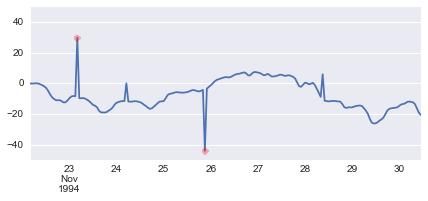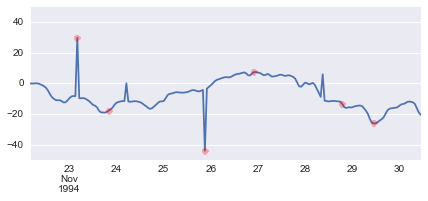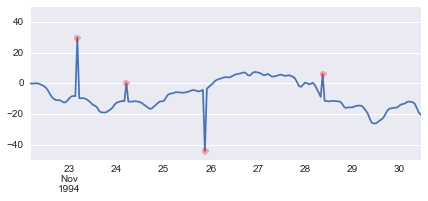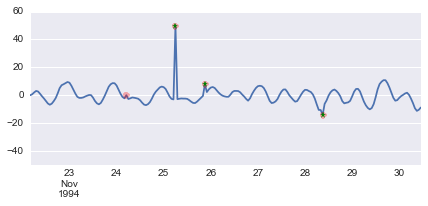# 3 ways to remove outliers from your data

According to Google Analytics, my post "Dealing with spiky data", is by far the most visited on the blog. I think that the reasons are: it is one of the oldest posts, and it is a real problem that people have to deal everyday.

Recently I found an amazing series of post writing by Bugra on how to perform outlier detection using FFT, median filtering, Gaussian processes, and MCMC

I will test out the low hanging fruit (FFT and median filtering) using the same data from my original post.

In :
from datetime import datetime

fname = './data/spikey_v.dat'
cols = ['j', 'u', 'v', 'temp', 'sal', 'y', 'mn', 'd', 'h', 'mi']

df = read_table(fname , delim_whitespace=True, names=cols)

df.index = [datetime(*x) for x in zip(df['y'], df['mn'], df['d'], df['h'], df['mi'])]
df = df.drop(['y', 'mn', 'd', 'h', 'mi'], axis=1)


Out:
j u v temp sal
1994-11-22 04:00:00 1056.1667 -0.1 0.0 23.9 34.6
1994-11-22 05:00:00 1056.2083 -0.2 0.7 23.9 34.6
1994-11-22 06:00:00 1056.2500 -0.1 2.0 23.9 34.6
1994-11-22 07:00:00 1056.2917 0.0 3.1 23.9 34.6
1994-11-22 08:00:00 1056.3333 -0.1 2.7 23.9 34.6

Starting with Bugra's get_median_filtered() we have:

In :
import numpy as np

def get_median_filtered(signal, threshold=3):
signal = signal.copy()
difference = np.abs(signal - np.median(signal))
median_difference = np.median(difference)
if median_difference == 0:
s = 0
else:
s = difference / float(median_difference)
return signal

In :
import matplotlib.pyplot as plt

figsize = (7, 2.75)
kw = dict(marker='o', linestyle='none', color='r', alpha=0.3)

df['u_medf'] = get_median_filtered(df['u'].values, threshold=3)

outlier_idx = np.where(df['u_medf'].values != df['u'].values)

fig, ax = plt.subplots(figsize=figsize)
df['u'].plot()
df['u'][outlier_idx].plot(**kw)
_ = ax.set_ylim(-50, 50)Not bad. Still, it missed the two lower spikes. Let's go for the detect_outlier_position_by_fft().

In :
def detect_outlier_position_by_fft(signal, threshold_freq=0.1,
frequency_amplitude=.001):
signal = signal.copy()
fft_of_signal = np.fft.fft(signal)
outlier = np.max(signal) if abs(np.max(signal)) > abs(np.min(signal)) else np.min(signal)
if np.any(np.abs(fft_of_signal[threshold_freq:]) > frequency_amplitude):
index_of_outlier = np.where(signal == outlier)
return index_of_outlier
else:
return None

In :
outlier_idx = []

y = df['u'].values

opt = dict(threshold_freq=0.01, frequency_amplitude=0.001)

win = 20
for k in range(win*2, y.size, win):
idx = detect_outlier_position_by_fft(y[k-win:k+win], **opt)
if idx is not None:
outlier_idx.append(k + idx - win)
outlier_idx = list(set(outlier_idx))

fig, ax = plt.subplots(figsize=(7, 2.75))

df['u'].plot()
df['u'][outlier_idx].plot(**kw)
_ = ax.set_ylim(-50, 50)Not sure if this method is the best here... Maybe if the signal was contaminated by high frequency noise this method would perform better.

Inspired by Bugra's median filter let's try a rolling_median filter using pandas.

In :
from pandas import rolling_median

threshold = 3
df['pandas'] = rolling_median(df['u'], window=3, center=True).fillna(method='bfill').fillna(method='ffill')

difference = np.abs(df['u'] - df['pandas'])
outlier_idx = difference > threshold

fig, ax = plt.subplots(figsize=figsize)
df['u'].plot()
df['u'][outlier_idx].plot(**kw)
_ = ax.set_ylim(-50, 50)Update: A friend, that knows this data, challenged me to use the same technique on $v$. Here it is:

In :
df['pandas'] = rolling_median(df['v'], window=3, center=True).fillna(method='bfill').fillna(method='ffill')

difference = np.abs(df['v'] - df['pandas'])
outlier_2 = difference > 2
outlier_3 = difference > 3

fig, ax = plt.subplots(figsize=figsize)
df['v'].plot()
df['v'][outlier_2].plot(**kw)
kw.update(color='g', marker='*', alpha=1)
df['v'][outlier_3].plot(**kw)
_ = ax.set_ylim(-50, 60)Note that I had to reduce the threshold from 3 -> 2 to get them all.

In :
HTML(html)

Out:

This post was written as an IPython notebook. It is available for download or as a static html.